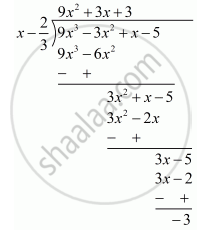Advertisement Remove all ads

# f(x) = 9x3 − 3x2 + x − 5, g(x) = x − 2 3 - Mathematics

Answer in Brief

f(x) = 9x3 − 3x2 + x − 5, g(x) = $x - \frac{2}{3}$

Advertisement Remove all ads

#### Solution

Let us denote the given polynomials as

f(x) = 9x^2 - 3x^2 + x -5

g(x) = x2/3

We have to find the remainder when f(x) is divided by g(x).

By the remainder theorem, when f(x) is divided by g(x) the remainder is

f(2/3) = 9(2/3)^2 - 3 (2/3)^2 + (2/3) - 5

= 9 xx 8 /27 - 3 xx 4/9 + 2/3 - 5

 = 8/3 - 4/3 + 2/3 - 5

= -3

Remainder by actual divisionRemainder is −3

Is there an error in this question or solution?
Advertisement Remove all ads

#### APPEARS IN

RD Sharma Mathematics for Class 9
Chapter 6 Factorisation of Polynomials
Exercise 6.3 | Q 7 | Page 14
Advertisement Remove all ads

#### Video TutorialsVIEW ALL 

Advertisement Remove all ads
Share
Notifications

View all notifications

Forgot password?
Course